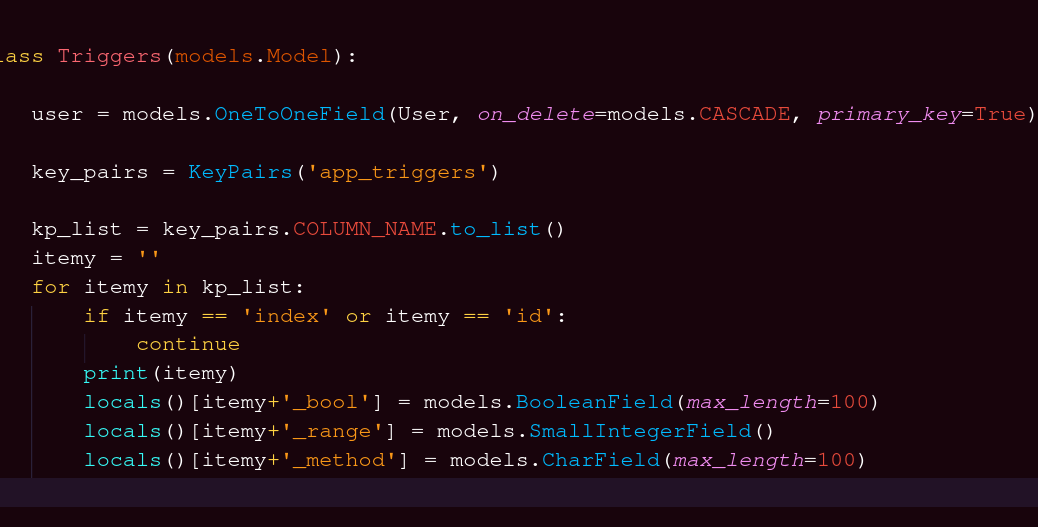# Advanced Django Tricks: Dynamically & Automatically Generate Form Fields Using Python Local Variables

The following is a trick I use to dynamically generate form fields before you know the form column names.

This allows you to:

– dynamically populate form fields based on database columns
– editing database columns will automatically & dynamically add or remove the columns from your form

Brief overview:

OLD CODE:

```class UserExtras(models.Model):
name = models.CharField(max_length=100)
age = models.CharField(max_length=100)
website = models.CharField(max_length=100)
height = models.CharField(max_length=100)
weight = models.CharField(max_length=100)
shoe_size = models.CharField(max_length=100)```

NEW CODE:

```class UserExtras(models.Model):
db_columns = ['name', 'age', 'website', 'height', 'weight', 'shoe_size']
for db_col in db_columns:
if db_columns == 'index' or db_columns == 'id':
continue
locals()[db_col] = models.CharField(max_length=100)```

What we are doing it adding the fields dynamically to the local variables inside our object

We can skip this by calling the table’s fields as these strings. Because these model objects do not include self, they cannot use setattr.

However, you can manually add them to locals()[array].

### Consider this additional django form multiple fields example:

models.py

```from django.db import models
from django.contrib.auth.models import User
from django.db import connections
from django.conf import settings

class UserTop(models.Model):
email = models.CharField(max_length=100, blank=True, null=True)
first_name = models.CharField(max_length=100, blank=True, null=True)
last_name = models.TextField(blank=True, null=True)
class Meta:
db_table = 'auth_user'```

views.py

```from django.forms import *
from app.models import *
from .forms import *

def user_top(request):
msg = None
if request.method == 'POST':
form = UserUpdateForm(request.POST)
# id = UserTop.objects.get(id=request.user).user
if form.is_valid():
id = form.cleaned_data.get('id')
email = form.cleaned_data.get('email')
first_name = form.cleaned_data.get('first_name')
last_name = form.cleaned_data.get('last_name')
p = UserTop(
id=id,
email=email,
first_name=first_name,
last_name=last_name
)
p.save()
msg = 'Saved.'
return HttpResponse(json.dumps({'msg': msg}), content_type='application/json')
else:
msg = 'Form is not valid'
else:
form = ProfileForm()
return render(msg, 'profile.html', {'form': form})

```

forms.py

```class UserUpdateForm(forms.Form):
id = forms.CharField(widget=forms.TextInput(attrs={"placeholder" : "Id","class": "form-control"}))
email = forms.EmailField(widget=forms.EmailInput(attrs={"placeholder" : "Email","class": "form-control"}))
first_name = forms.CharField(widget=forms.EmailInput(attrs={"placeholder" : "First Name","class": "form-control"}))
last_name = forms.CharField(widget=forms.EmailInput(attrs={"placeholder" : "Last Name","class": "form-control"}))
class Meta:
model = Userfields = ('username', 'email', 'first_name', 'last_name')
```

urls.py

```    path('edit-user-master/', views.user_top, name='profile.html'),
```

In this example we have a working custom form that allows a user to change his/her username, email, first_name and last_name.

Note the class Meta: db_table = ‘auth_user’ reference on the models.py. We can use forms.ModelForm but when we use forms.Form we must add the class Meta table name.

# Magic Django Automatic Dynamic Form Field Generator

ADD TO THE TOP OF models.py

```def KeyPairs(arg):
from django.db import connection
import pandas as pd
cursor = connection.cursor()
"SELECT TABLE_NAME, COLUMN_NAME FROM `information_schema`.`COLUMNS` \
WHERE CONVERT(`TABLE_NAME` USING utf8) LIKE '%"+arg+"%'",
connection
)
return df```

This function method will query your Database a return a pandas dataframe of your Column Names.

Now consider this replacement to the class UserTop(models.Model) at the start of this demonstration:

models.py

```class UserTop(models.Model):
key_pairs = KeyPairs('auth_user')
kp_list = key_pairs.COLUMN_NAME.to_list()
itemy = ''
for column_name in kp_list:
# set columns to ignore here
if column_name == 'email' or column_name == 'user_id':
continue
if column_name == 'bio':
locals()[itemy] = models.TextField(blank=True, null=True)
else:
locals()[itemy] = models.CharField(max_length=100)
class Meta:
db_table = 'auth_user'```

# Add many 200+ Form Fields automatically in Django go in this example:

THIS:

BECOMES THIS!!

models.py

```def KeyPairs(arg):
from django.db import connection
import pandas as pd
cursor = connection.cursor()
"SELECT TABLE_NAME, COLUMN_NAME FROM `information_schema`.`COLUMNS` \
WHERE CONVERT(`TABLE_NAME` USING utf8) LIKE '%"+arg+"%'",
connection
)
return df

class Triggers(models.Model):
user = models.OneToOneField(User, on_delete=models.CASCADE, primary_key=True) #, related_name='user'
key_pairs = KeyPairs('app_triggers')
kp_list = key_pairs.COLUMN_NAME.to_list()
itemy = ''
for itemy in kp_list:
if itemy == 'index' or itemy == 'id':
continue
locals()[itemy+'_bool'] = models.BooleanField(max_length=100)
locals()[itemy+'_range'] = models.SmallIntegerField()
locals()[itemy+'_method'] = models.CharField(max_length=100)```

Just to demonstrate how powerful this is, I used it to add 200+ form fields.